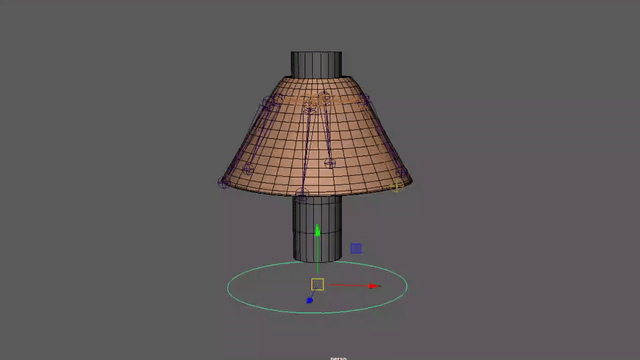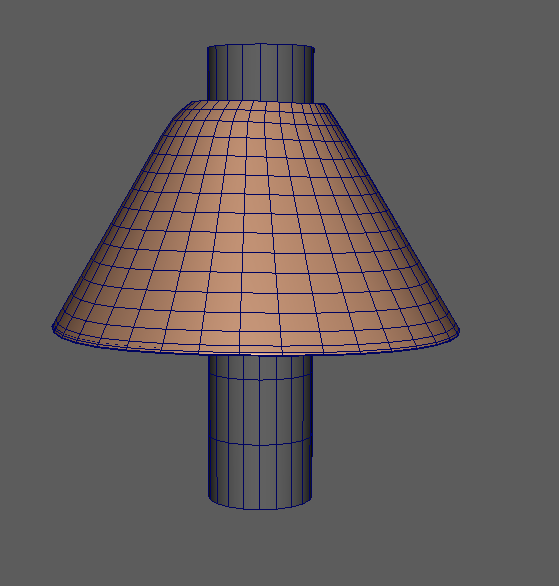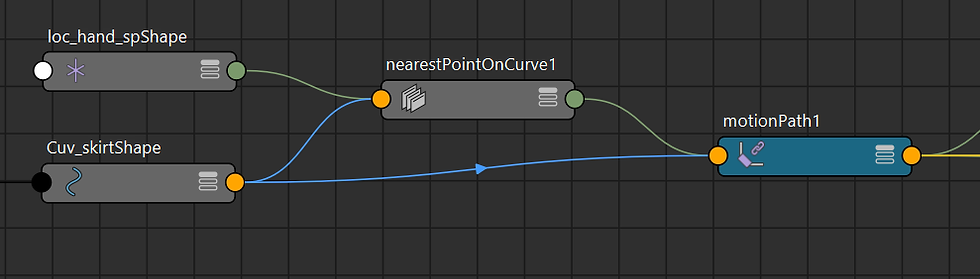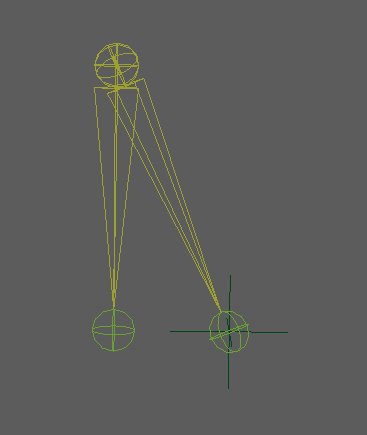top of page
Search
• Liling Liu

# How to Create A Joint-Based Skirt Rig With Auto Collision In The Maya

Updated: Sep 3Due to the collision between the two objects, they can be used to generate interaction. The animator can quickly and easily make the animation and it can be export them into the game engine. My thoughts that want to use this ideas and technique to make the skirt rig. This rig is base on joints and it is suitable for the game animation

In this tutorial, I will use simple object and skirt model to rig.

I will break the post into 4 main sections.

1. Calculate the distance between an object and the circle

2. Calculate the distance between skirt and the circle

3. Determine the distance between object and skirt to collide

4. Calculate the angle of the object to collide

Before I start the tutorial, I would like to talk concept principle

The concept is during of letting object one collides with another object two, they start to collide. First, make a circle which simply represents the structure of the skirt into inside the skirt. Second, when the object one is moving, need to know how the distance and angle are between with the circle point. Using this angle to collide object two. Also, it needs to know how the object two and circle point’s distance to get the collision.

## Calculate the distance between an object and the circleFirst, create bones to each object and a circle curve. Shape the curve along the inside of the skirt. Since you want to know how far the object is from the circle when moving. Create a Locator (name: loc_hand_sp) and parent under the long object bone. Use this Locator to calculate with the point on the circle. Before calculating, you must know how the point value on the circle currently.

Create a Locator (name: loc_cuv) and add a circle line to do attach to motion path. Go to Constrain> Motion Paths> Attach to Motion Path. In the Motion Path Attribute, you can see that the U Value is locked. At this time, you can unlock it, and you can see the locator movement on the circle when you move the U Value

Calculate the points of loc_hand_sp and the circle line (Cuv_skirt):

1. Go to Windows> Node Editor in Maya and create the NearestPointOnCurve node in the editor.

2. Connect loc_hand_spShape worldPosition to the NearestPointOnCurve node in position

Connect Cuv_skirtShape's worldspace to the NearestPointOnCurve input curve

3. Then connect the NearestPointOnCurve parameter to the motionpath U ValueIf you are not familiar with Maya node, you can go to Autodesk page. https://knowledge.autodesk.com/support/maya/learn-explore/caas/CloudHelp/cloudhelp/2019/ENU/Maya-LightingShading/files/GUID-DA9707D2-8A0D-4911-A010-8274C57D3FD3-htm.html

## Calculate the distance between skirt and the circleGo to Create > Measure Tools > Distance Tool.

Measure the distance between the bones of the skirt and the locator (loc_cuv) on the circle point

## Determine the distance between object and skirt to collide

• Create 2 multiplyDivide in node editor to calculate the distance. These two nodes operation change to Divide. Connect The distance line (DD_L_skirt_02_DistShape) distance to the multiplyDivide1 input2 and Input1 sets to 1. Connect multiplyDivide1 output to the multiplyDivide2 input 1, and copy the value of input1 to input 2.

• After obtaining this value, it is necessary to determine whether the value is within the distance. If the value is within the distance, it can be changed. If it is not, keep it in place. Create a remapValue. This node can be placed to determine the value, and the maximum and minimum values set by yourself. If the number is between, it starts to produce the calculation result. If the value is not between, it outputs the desired of maximum and the minimum value. Here, set the minimum value is 0 and the maximum value is 1. Connect multiplyDivide2 output to the remapValue input value, and adjust the input min and max value.## Calculate the angle of the object to collide

Create a new multiplyDivide. Connect remapValue output to the multiplyDivide input1 (multiplyDivide3). Connect multiplyDivide3 output to the skirt bone. Later, will use multiplyDivide3 input 2 as the angle of the object. If the angle exceeds a value, it can start to collide and rotate the bones of the skirt. Repeat the about step for every skirt bones.Determine angle:• Create two sets of bones, and each of the bottom bones place on the locator. Then, one set of bones (straight) parent under the another. The bone rotation value will automatically turn into joint orient. Copy the joint orient number to the rotation, so that you can know the angle of these two groups of bones.

• Create IK Handle to the bone (oblique) and it parents into locator on the circle point.

• Select the loc_hand_sp and the bone (straight) to do aim constraint option. In the window, set the world up type to Object rotation up and put bone (oblique) names on it. Also, set the up vector and world up vector the value that it depends locator world axis. As long as the two bones meet again, the angle will be 0. If the angle is exceeded, the skirt bones will be rotated.

• Create clamp node. Connect the bone (straight) to the clamp inputR , and adjust the MaxR value. If the angle is between this, the output is 0; otherwise, it is 1. Connect clamp output R to the multiplyDivide3 input 2

• In order to adjust how much the angle rotate, create a multiplyDivide. Connect multiplyDivide3 output to the multiplyDivide4 input1. Now, adjust multiplyDivide4 input2 value to get the rotation what we want. Select the controller and create the attribute so as to adjust the value. Connect the attribute to the multiplyDivide4 input2.

17,235 views
bottom of page